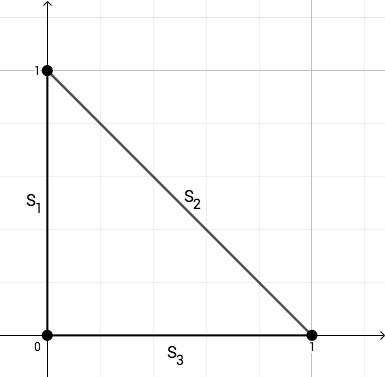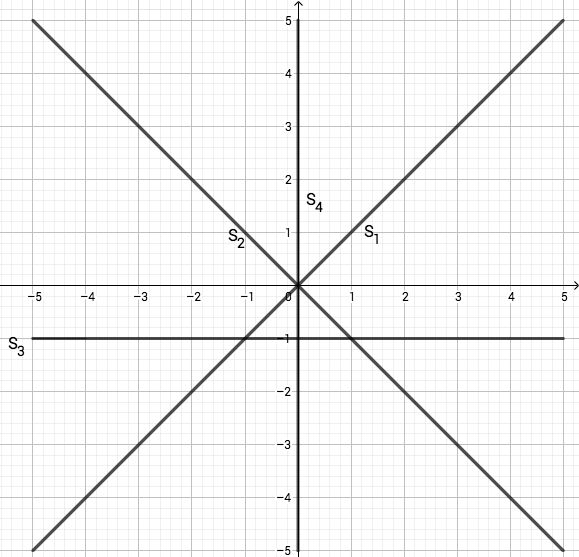시간 제한 메모리 제한 제출 정답 맞은 사람 정답 비율
2 초 512 MB 1 1 1 100.000%

## 문제

Jaehyun likes computational geometry. Here is Jaehyun's question: "We are given $n$ segments on the Cartesian plane. Count the number of simple cycles in the generated graph."

Formally, a set of $n$ segments $S = \{s_1, s_2, \ldots, s_n\}$ generates the following graph $G = (V, E)$.

For a point $v$ of the plane, $v \in V$ if $v$ is one of the endpoints of the segments or $v$ is an intersection of two or more segments.

For two distinct vertices $u$ and $v$, $(u, v) \in E$ if there is a segment $s_i \in S$ containing vertices $u$ and $v$, and there is no vertex on $s_i$ between $u$ and $v$.

A simple cycle is a cycle with no repeated vertices or edges.

Zigui tried to solve Jaehyun's problem, and he found it is possible to make various answers with just a few segments.

For given $N$, find a set of segments such that the number of simple cycles in the graph generated by these segments is $N$.

## 입력

The first line contains an integer $N$ ($1 \le N \le 1000$).

## 출력

The first line of the output must contain an integer $K$: the number of segments ($1 \le K \le 12$).

Each of the next $K$ lines must contain four integers $x_{1}$, $y_{1}$, $x_{2}$, and $y_{2}$ denoting a segment with endpoints $(x_{1}, y_{1})$ and $(x_{2}, y_{2})$ ($-10^{9} \le x_{1}, y_{1}, x_{2}, y_{2} \le 10^{9}$, $(x_{1}, y_{1}) \neq (x_{2}, y_{2})$).

## 예제 입력 1

1


## 예제 출력 1

3
0 0 0 1
1 0 0 1
1 0 0 0## 예제 입력 2

3


## 예제 출력 2

4
-5 -5 5 5
-5 5 5 -5
-5 -1 5 -1
0 -5 0 5